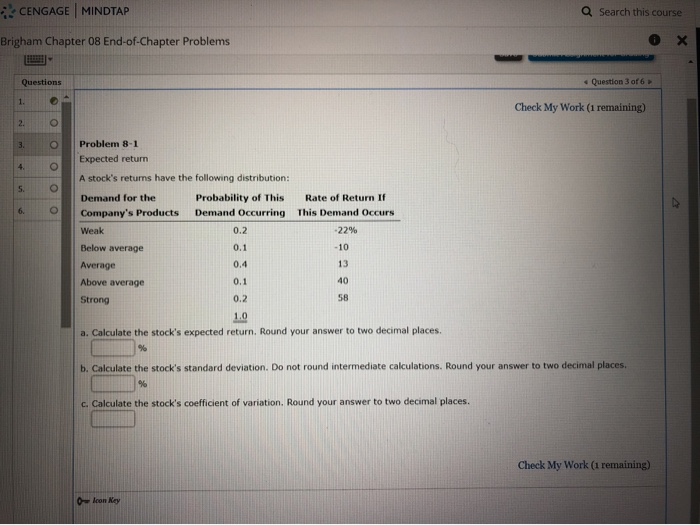# Q Search this course CENGAGE | MINDTAP Brigham Chapter 08 End-of-Chapter Problems 0 x Question 3 of 6 Check My Wo...Q Search this course CENGAGE | MINDTAP Brigham Chapter 08 End-of-Chapter Problems 0 x Question 3 of 6 Check My Work (1 remaining) Problem 8-1 Expected return A stock's returns have the following distribution: Demand for the Probability of this Rate of Return If Company's Products Demand Occurring This Demand Occurs Weak .22% Below average Average Above average Strong a. Calculate the stock's expected return, Round your answer to two decimal places. b. Calculate the stock's standard deviation. Do not round intermediate calculations. Round your answer to two decimal places c. Calculate the stock's coefficient of variation. Round your answer to two decimal places. Check My Work (1 remaining) 0 loon Key

Ans a - Expected Return is 15.40%

 Demand of Product Probability Rate of Returns Probability * Returns Weak 0.2 -22% -4.40% Below Average 0.1 -10% -1.00% Average 0.4 13% 5.20% Above Average 0.1 40% 4.00% Strong 0.2 58% 11.60% Expected Return 15.40%

Ans b - Standard Deviation 27.75

Demand of Product Probability Rate of Returns Probability * Returns
 X - mean
 p*(X - mean)^2
Weak 0.2 -22 -4.4 -37.4 279.752
Below Average 0.1 -10 -1 -25.4 64.516
Average 0.4 13 5.2 -2.4 2.304
Above Average 0.1 40 4 24.6 60.516
Strong 0.2 58 11.6 42.6 362.952
Expected Return 15.4 770.04
Variance 770.04
SD 27.75

SD is squire root of variance.

Ans - 3 Coefficient of variance is SD / Mean

= 27.75 / 15.40 = 1.80

Hope this helps.

##### Add Answer of: Q Search this course CENGAGE | MINDTAP Brigham Chapter 08 End-of-Chapter Problems 0 x Question 3 of 6 Check My Wo...
More Homework Help Questions Additional questions in this topic.

• #### MindTap- Cengage Learning -Mozilla Firefox ⓘ https://ng cengage.com stat /nbu evo/index.html?depl...

Need Online Homework Help?